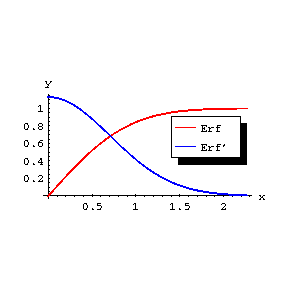# Probability integral

error integral

The function

$$\mathop{\rm erf} ( x) = \ \frac{2}{\sqrt \pi } \int\limits _ { 0 } ^ { x } e ^ {- t ^ {2} } d t ,\ \ | x | < \infty .$$

In probability theory one mostly encounters not the probability integral, but the normal distribution function

$$\Phi ( x) = \ \frac{1}{\sqrt {2 \pi } } \int\limits _ {- \infty } ^ { x } e ^ {- t ^ {2} / 2 } d t = \frac{1}{2} \left [ 1 + \mathop{\rm erf} \left ( \frac{x}{\sqrt 2 } \right ) \right ] ,$$

which is the so-called Gaussian probability integral. For a random variable $X$ having the normal distribution with mathematical expectation 0 and variance $\sigma ^ {2}$, the probability that $| X | \leq t$ is equal to $\mathop{\rm erf} ( t / \sqrt 2 )$. For real $x$, the probability integral takes real values; in particular,

$$\mathop{\rm erf} ( 0) = 0 ,\ \ \lim\limits _ {x \rightarrow + \infty } \mathop{\rm erf} ( x) = 1 .$$Figure: p074920a

The graph of the probability integral and its derivatives are illustrated in the figure. Regarded as a function of the complex variable $z$, the probability integral $\mathop{\rm erf} ( z)$ is an entire function of $z$.

The asymptotic representation for large $z$, $\mathop{\rm Re} z > 0$, is given by:

$$1 - \mathop{\rm erf} ( z) \sim \ \frac{e ^ {- z ^ {2} } }{\sqrt {\pi z } } \left ( 1 + \sum _ { k= } 1 ^ \infty ( - 1 ) ^ {k} \frac{1 \cdot 3 \dots ( 2 k - 1 ) }{2 ^ {k} } \frac{1}{z ^ {2k} } \right ) .$$

In a neighbourhood of $z = 0$ the probability integral can be represented by the series

$$\mathop{\rm erf} ( z) = \ \frac{2}{\sqrt \pi } \left ( z - \frac{z ^ {3} }{1!3} + \dots + \frac{( - 1 ) ^ {k} }{k ! ( 2 k + 1 ) } z ^ {2k+} 1 + \dots \right ) .$$

The probability integral is related to the Fresnel integrals $C ( z)$ and $S ( z)$ by the formulas

$$1+ \frac{i}{2} \ \mathop{\rm erf} \left ( 1- \frac{i}{\sqrt 2} z \right ) = \ C ( z) + i S ( z) ,$$

$$1- \frac{i}{2} \mathop{\rm erf} \left ( 1+ \frac{i}{\sqrt 2} z \right ) = C ( z) - i S ( z) .$$

The derivative of the probability integral is given by:

$$[ \mathop{\rm erf} ( z) ] ^ \prime = \ \frac{2}{\sqrt \pi} e ^ {- z ^ {2} } .$$

The following notations are sometimes used:

$$\Theta ( x) = H ( x) = \ \Phi ( x) = \mathop{\rm erf} ( x) ,$$

$$\mathop{\rm Erf} ( x) = \frac{\sqrt \pi }{2} \mathop{\rm erf} ( x) ,$$

$$\mathop{\rm Erfi} ( x) = - i \frac{\sqrt \pi }{2} \mathop{\rm erf} ( i x ) = \int\limits _ { 0 } ^ { x } e ^ {t ^ {2} } d t ,$$

$$\mathop{\rm Erfc} ( x) = \frac{\sqrt \pi }{2} - \mathop{\rm Erf} x = \int\limits _ { x } ^ \infty e ^ {- t ^ {2} } d t ,$$

$$\alpha ( x) = \frac{2}{\sqrt \pi} \int\limits _ {- \infty } ^ { x } e ^ {- t ^ {2} } d t - 1 = \frac{2} \pi \mathop{\rm Erf} \left ( \frac{x}{\sqrt 2} \right ) .$$

How to Cite This Entry:
Probability integral. Encyclopedia of Mathematics. URL: http://encyclopediaofmath.org/index.php?title=Probability_integral&oldid=48299
This article was adapted from an original article by A.B. Ivanov (originator), which appeared in Encyclopedia of Mathematics - ISBN 1402006098. See original article